Andersen theorem

A result in the theory of fluctuations in random walks (cf. Random walk). Let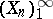be independent random variables with the same distribution (cf. Random variable), and let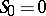,,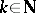. Define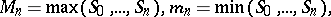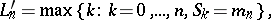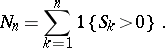Then (equivalence principle) for each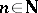the pairs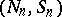,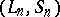and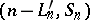have the same distribution; in particular,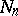,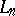and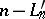have the same distribution. As a consequence one has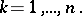These results were first proved by E. Sparre Andersen [a1], [a2], [a3]. They connect the arcsine law for random walks to the arcsine law in renewal theory.

Nowadays there are brief proofs based on combinatorial properties of non-random sequences [a6], [a7]. The results can be generalized to random vectors with symmetric distributions [a2]. A comprehensive account for integer-valued random variables can be found in [a8]; a concise overview is given in [a4]. Related combinatorial results are discussed in [a5].